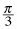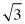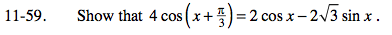Home > PC > Chapter 11 > Lesson 11.1.4 > Problem11-59

11-59.

Show that 4 cos (x +) = 2 cos x − 2sin x. Homework Help ✎Use the Angle Sum Identity.

$4\left( \cos(\textit{x})\cos\left( \frac{\pi}{3} \right) - \sin(\textit{x})\sin\left( \frac{\pi}{3} \right)\right) =$

Substitute the exact values for sine and cosine of π/3. Then simplify.